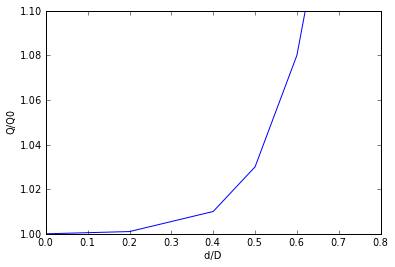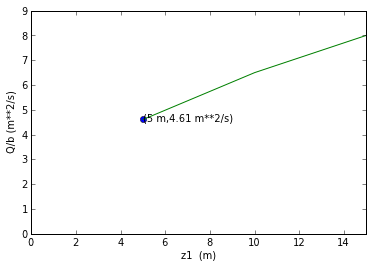# Chapter 3 :Elementary fluid dynamics¶

### Example 3.1 Page No.99¶

In :
v1=100                            #mi/hr
ht=10000                         #ft
#from standard table for static pressure at an altitude
p1=1456                        #lb/ft**2(abs)
P1=1456*0.006947        #psi
d=0.001756                   #slugs/ft**3
#1 mi/hr = 1.467 ft/s

#calculation
p2=p1+(d*(v1*1.467)**2/2)          #lb/ft**3
#in terms of gage pressure p2g
p2g=p2-p1                                   #lb/ft**2
#1lb/ft**2 = 0.006947 psi
P2=p2*0.006947                         #psi
P2g=p2g*0.006947#psi
#pressure difference indicated by the pitot tube = pdiff
pdiff=P2-P1                               #psi

#Result
print "Pressure at point 1 =",round(P1,2),"psi"
print "Pressure at point 2 in terms of gage pressure=",round(P2g,2),"psi"
print "pressure difference indicated by the pitot static tube=",round(pdiff,2),"psi"

Pressure at point 1 = 10.11 psi
Pressure at point 2 in terms of gage pressure= 0.13 psi
pressure difference indicated by the pitot static tube= 0.13 psi


### Example 3.7 Page No.115¶

In :
%matplotlib inline

Welcome to pylab, a matplotlib-based Python environment [backend: module://IPython.zmq.pylab.backend_inline].

In :
dia=0.1                             #m
dia1=1.0                           #m
h=2.0                               #m

#calculation
#bernoulli's equation: p1+(0.5*d*V1**2)+(sw*z1)= p2+(0.5*d*V2**2)+(sw*z2)
#assuming p1=p2=0, and z1=h and z2=0
#(0.5*d*V1**2)+(g*h)= (0.5*d*V2**2)
#assuming steady flow Q1=Q2, Q=A*V. hence, A1*V1=A2*V2
#V1=((dia/dia1)**2)*V2
#hence V2=((2*g*h)/(1-(dia/dia1)**4))**0.5
import math
V2=((2*9.81*h)/(1-(dia/dia1)**4))**0.5
Q=(math.pi/4*(dia)**2)*V2

#result
print "The flow rate needed is=",round(Q,5),"m**3/s"

#Plot
h=[0,0.2,0.4,0.5,0.6,0.62]
D=[1,1.001,1.01,1.03,1.08,1.10]
xlabel("d/D ")
ylabel("Q/Q0")
plt.xlim((0,0.8))
plt.ylim((1,1.10))
a=plot(h,D)
show(a)

The flow rate needed is= 0.0492 m**3/s### Example 3.8 Page No.116¶

In :
dia=0.03          #m
dia1=0.01        #m
p=3                 #kPa(gage)

#calculation
#density of air d is found using standard temp and pressure conditions
d=(p+101)*1000/((286.9)*(15+273))
#applying Bernoulli's equation at points 1,2 and 3 p=p1
import math
v3=((2*p*1000)/d)**0.5
Q=(math.pi)/4*(dia1**2)*v3
#by continuity equation, A2*v2=A3*v3
v2=((dia1/dia)**2)*v3
p2=(p*1000)-(0.5*d*(v2**2))

#Result
print  "Flowrate =",round(Q,5),"m**3/s"
print "Pressure in the hose=",round(p2,1),"N/m**2"

Flowrate = 0.00542 m**3/s
Pressure in the hose= 2963.0 N/m**2


### Example 3.10 Page No. 120¶

In :
T=60                               #degree farenheit
z1=5                               #ft
atmp=14.7                      #psia
#applying bernoulli equation at points 1,2 and 3
z3=-5                            #ft
v1=0                             #large tank
p1=0                             #open tank
p3=0                             #open jet
#applying continuity equation A2*v2=A3*v3 A2=A3 so v2=v3

#calculation
v3=(2*32.2*(z1-z3))**0.5
#vapor pressure of water at 60 degree farenheit = p2=0.256 psia
p2=0.256
z2=z1-((((p2-atmp)*144)+(0.5*1.94*v3**2))/62.4)

#result
print "The maximum height over which \n the water can be siphoned without cavitation occuring=",round(z2,3),"ft"

The maximum height over which
the water can be siphoned without cavitation occuring= 28.321 ft


### Example 3.11 Page No.122¶

In :
sg=0.85
Q1=0.005                        #m**3/s
Q2=0.05                         #m**3/s
dia1=0.1                         #m
dia2=0.06                        #m

#calculation
#A2/A1=dia2/dia1
import math
d=sg*1000
Arat=(dia2/dia1)**2
A2=math.pi/4*(dia2**2)
pdiffs=(Q1**2)*d*(1-(Arat**2))/(2*1000*(A2**2))
pdiffl=(Q2**2)*d*(1-(Arat**2))/(2*1000*(A2**2))
print "The pressure difference ranges from =",round(pdiffs,3),"kpa"  "to" ,round(pdiffl,0),"kpa"

The pressure difference ranges from = 1.157 kpato 116.0 kpa


### Example 3.12 Page No.124¶

In :
z1=5                          #m
a=0.8                        #m
b=6                          #m
Cc=0.61                 #since a/z1=ratio=0.16<0.2 Cc= contracction coefficient
z2=Cc*a

#calculation
#Q/b=flowrate
flowrate=z2*((2*9.81*(z1-z2))/(1-((z2/z1)**2)))**0.5
#considering z1>>z2 and neglecting kinetic energy of the upstream fluid
flowrate1=z2*(2*9.81*z1)**0.5
#result
print "The flowrate per unit width=",round(flowrate,2),"m**2/s"
print "The flowrate per unit width when we consider z1>>z2=",round(flowrate1,1),"m**2/s"

#Plot
import matplotlib.pyplot as plt
fig = plt.figure()

h=[5,10,15]
D=[4.61,6.5,8]
xlabel("z1  (m)")
ylabel("Q/b (m**2/s)")
plt.xlim((0,15))
plt.ylim((0,9))

ax.plot(, [4.61], 'o')
ax.annotate('(5 m,4.61 m**2/s)', xy=(5,4.5))
a=plot(h,D)
show(a)

The flowrate per unit width= 4.61 m**2/s
The flowrate per unit width when we consider z1>>z2= 4.8 m**2/s### Example 3.13 Page No.125¶

In :
Qrat=3**2.5

#Result
print("The flowrate is proportional to H**2.5")
print "When depth is increased from H0 to 3H0 Q increases ",round(Qrat,1),"times"

The flowrate is proportional to H**2.5
When depth is increased from H0 to 3H0 Q increases  15.6 times


### Example 3.15 Page No.130¶

In :
h=10                       #Km
#air is in a standard atmosphere
p1=26.5                 #kPa
T1=-49.9               #degree celcius
d=0.414                #Kg/m**3
k=1.4
Ma1=0.82             #Mach
#for incompressible flow,
pdiff=(k*Ma1**2)/2*p1
#for compressible isentropic flow,
pdiff1=((1+((k-1)/2)*(Ma1**2))**(k/(k-1))-1)*p1

#result
print("Stagnation pressure on leading edge on the wing of the Boeing:")
print "when flow is imcompressible =",round(pdiff,1),"kpa"
print "when flow is compressible and isentropic =",round(pdiff1,1),"kpa"

Stagnation pressure on leading edge on the wing of the Boeing:
when flow is imcompressible = 12.5 kpa
when flow is compressible and isentropic = 14.7 kpa


### Example 3.17 Page No.132¶

In :
V=5               #m/s
sg=1.03
h=50              #m

p2=(((sg*1000)*(V**2)/2) + (sg*1000*9.81*h))/1000  #kPa
print "The pressure at stagnation point 2 =",round(p2,1),"kpa"

The pressure at stagnation point 2 = 518.1 kpa